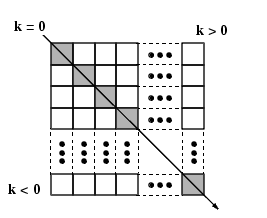MATLAB Function Referencediag

Diagonal matrices and diagonals of a matrix

Syntax

• ```X = diag(v,k)
X = diag(v)
v = diag(X,k)
v = diag(X)
```

Description

```X = diag(v,k) ``` when `v` is a vector of `n` components, returns a square matrix `X` of order `n+abs(k)`, with the elements of `v` on the `k`th diagonal. `k = 0` represents the main diagonal, `k > 0` above the main diagonal, and `k < 0` below the main diagonal.```X = diag(v) ``` puts `v` on the main diagonal, same as above with `k` `=` `0`.

```v = diag(X,k) ``` for matrix `X`, returns a column vector `v` formed from the elements of the `k`th diagonal of `X`.

```v = diag(X) ``` returns the main diagonal of `X`, same as above with `k` `=` `0`.

Examples

`diag(diag(X))` is a diagonal matrix.

`sum(diag(X))` is the trace of `X`.

The statement

• ```diag(-m:m)+diag(ones(2*m,1),1)+diag(ones(2*m,1),-1)
```

produces a tridiagonal matrix of order `2*m+1`.

See Also

`spdiags`, `tril`, `triu`

© 1994-2005 The MathWorks, Inc.